Okay, let's see some examples! We'll create new databases from old using Kan extensions. You can see Fong and Spivak doing this in Section 3.4 of _[Seven Sketches](http://math.mit.edu/~dspivak/teaching/sp18/7Sketches.pdf)_, but I'll warn you that they don't say "Kan extension". They call the left Kan extension \$$\sum\$$ and the right Kan extension \$$\prod\$$, for reasons that will eventually become clear. (All mathematical concepts are connected.)

We'll start with the easiest example that's really nice. As a database schema let's use the free category on this graph: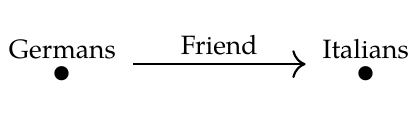Let's call this category \$$\mathcal{C}\$$. A functor \$$F: \mathcal{C} \to \mathbf{Set} \$$ is a database consisting of a set of Germans, a set of Italians, and a function mapping each German to an Italian. We can present it in table form, like this: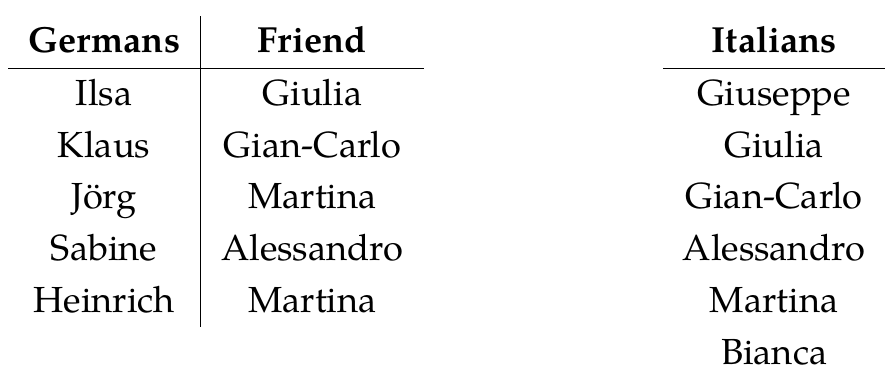Now suppose we want to forget all the information involving Italians. For this we need a simpler database schema, namely the free category on this graph: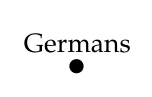Let's call this category \$$\mathcal{D}\$$. A functor \$$F: \mathcal{D} \to \mathbf{Set} \$$ is a database consisting of a set of Germans.

There's a unique functor

$G: \mathcal{D} \to \mathcal{C}$

with the property that

$G(\mathrm{Germans}) = \mathrm{Germans} .$

Any functor \$$F: \mathcal{C} \to \mathbf{Set} \$$ can be composed with \$$G\$$ to give a functor \$$F \circ G : \mathcal{D} \to \mathbf{Set} \$$. This process has the effect of forgetting the Italians!

So, if \$$F\$$ was this database:then \$$F \circ G\$$ is this database: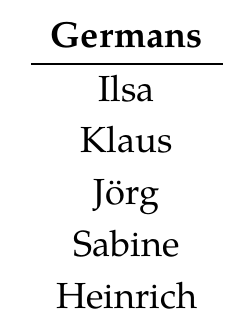Okay, that was pretty simple I hope! To put it in fancier terms, I just showed you what the functor

$\textrm{ composing with } G : \mathbf{Set}^{\mathcal{C}} \to \mathbf{Set}^{\mathcal{D}}$

does to objects - that is, databases. Now let's try to reverse this process, which is the fun part. Let's take a database that's just a set of Germans and try to turn it into a database that also includes Italians, and an Italian friend for every German!

Of course this is a bit odd, because we're trying to recover data that can't really be recovered. But that's exactly what adjoints are for.

There are two ways to proceed: the left adjoint way, called

$\textrm{Lan}_G : \mathbf{Set}^{\mathcal{D}} \to \mathbf{Set}^{\mathcal{C}}$

and the right adjoint way, called

$\textrm{Ran}_G : \mathbf{Set}^{\mathcal{D}} \to \mathbf{Set}^{\mathcal{C}}$

As usual, the left adjoint is 'liberal' or 'generous'. It says "We have to give each German an Italian friend? Okay, let's give them each their own friend!"

So, if we start with a database \$$H : \mathcal{D} \to \mathbf{Set} \$$ like this: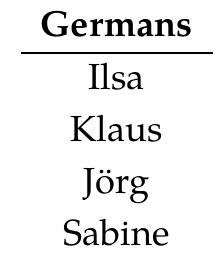then the left adjoint method gives us this database \$$\textrm{Lan}_G(H) : \mathcal{C} \to \mathbf{Set}\$$: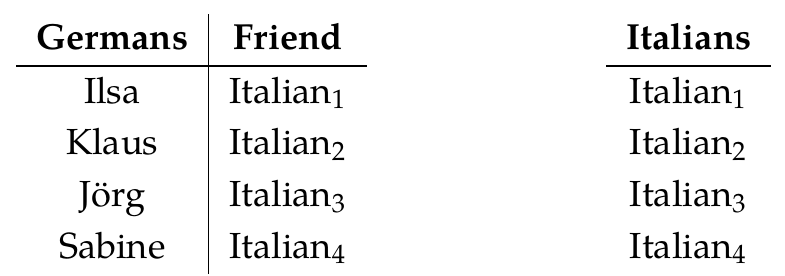In short, it makes up placeholders with arbitrary names to fill in the missing data. And it does this in a very systematic way. It doesn't add unnecessary equations, like giving Jörg and Sabine the same friend. And it doesn't add unnecessary elements, like some guy \$$\mathrm{Italian}_7\$$ who is not anybody's friend!

This is useful not because it gives us the 'right answer' to the impossible problem of guessing information about Italians when all we know about is the Germans. It's useful because it gives us an acceptable, systematically constructed database that we can then update as we learn more information.

The cool part is that all this follows from the definition of 'left adjoint'.

In other words, there's a natural one-to-one correspondence between

$\textrm{ morphisms from } \textrm{Lan}_G(H) \textrm{ to } F$

in the category \$$\mathbf{Set}^\mathcal{C}\$$ and

$\textrm{morphisms from } H \textrm{ to } F \circ G$

in the category \$$\mathbf{Set}^\mathcal{D}\$$.

Remember what these morphisms are: they are morphisms between functors, so they are _natural transformations_. Databases are functors, and we saw what natural transformations between them look like in [Puzzles 127-128](https://forum.azimuthproject.org/discussion/2244/lecture-43-chapter-3-natural-transformations/p1) and [Puzzle 148](https://forum.azimuthproject.org/discussion/2251/lecture-46-chapter-3-isomorphisms/p1).

So I'm saying that there's a natural one-to-one correspondence between

$\textrm{ natural transformations } \alpha: \textrm{Lan}_G(H) \Rightarrow F$

and

$\textrm{natural transformations } \beta: H \Rightarrow F \circ G$

This is true, not just for my particular example of \$$F\$$ and \$$H\$$, but for _any_ example. But let's think about it in my example.

**Puzzle 155.** Describe a natural transformation \$$\alpha : \textrm{Lan}_G(H) \Rightarrow F\$$ where \$$\textrm{Lan}_G(H) \$$ is this database:and \$$F\$$ is this database:**Puzzle 156.** Describe a natural transformation \$$\beta: H \Rightarrow F \circ G\$$ where \$$H \$$ is this database:and \$$F \circ G\$$ is this database:**Puzzle 157.** Which natural transformation \$$\beta: H \Rightarrow F \circ G\$$ corresponds to the natural transformation \$$\alpha :\textrm{Lan}_G(H) \Rightarrow F\$$ you gave in Puzzle 155?

**Puzzle 158.** How many natural transformations from \$$\textrm{Lan}_G(H)\$$ to \$$F\$$ are there? How many natural transformations from \$$H\$$ to \$$F \circ G\$$ are there?

You'd better get the same answer to both parts of Puzzle 158, since there's supposed to be a one-to-one correspondence between these natural transformations! However, it's best to think about both questions separately and see _why_ you're getting the same answer!

Finally:

**Puzzles 159.** The categories I've been calling \$$\mathcal{C}\$$ and \$$\mathcal{D}\$$ have other, more purely mathematical names. More precisely, they are _isomorphic_ to two other categories we've already seen in this course, which have more mathematical names. What are those other categories?

**[To read other lectures go here.](http://www.azimuthproject.org/azimuth/show/Applied+Category+Theory#Chapter_3)**